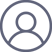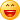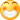吴中祥2019-10-19 07:46

客观世界统一的基本特性、运动规律(6)

((5))

由以*为中心变换到以‘为中心，相应的4维时空变矢量相应的可变系是：

r0’[0’]=r0*cA[0*]-r1*sA[1*]-r2*cB[2*]+r3*sB[3*]

r1’[1’]=r0*sA[0*]+r1*cA[1*]-r2*sB[2*]-r3*cB[3*]

r2’[2’]=r0*cB[0*]-r1*sB[1*]+r2* cA[2*]-r3*sA[3*]

r3[3’]’=r0*sB[0*]+r1*cB[1*]+r2*sA[2*]+r3* cA[3*] :

ra’[a’]={矩阵R(a’a*)ra*[a*],a*=03求和},a’ =03求和，

矩阵R(a’a*)=cA-sA-cB+sB

sA+cA-sB-cB

cB-sB+cA-sA

sB+cB+sa+cA，而有：

dr’[1线矢]=dra’[a’] a’ =03求和

={dR(a’a*)ra*[a*],a*=03求和},a’ =03求和

={dra’(偏分ra'R(a’a*))ra*[a*],a*=03求和},a’ =03求和

={dra’(w(a’a*)[a’])},a’ =03求和

= w(a’a*) dr*[1线矢]

w(a’a*)[a’]=(偏分ra'R(a’a*))ra*[a*],a*=03求和，

w(a’a*)=(偏分ra'R(a’a*))ra*,a*=03求和，是时空联络系数(Riemann-Christoffel符号)，具体表达了弯曲时空的基本特性。

3维空间矢量相应的可变系是：由3维空间的正交归一矩阵R(a’a*)联络系数(Riemann-Christoffel符号) w(a’a*)，表达。

(‘1)太阳系各行星的进动角

[进动角(1)]，并与其实测值[进动角(1)] Einstein理论值[进动角(1)] 比较(见下表)，（其中，冥王星现已被排除为大行星，但是，在此的有关数据，仍然有意义。）

天王  海王   冥王

P(0)=l(0)(1-e(0)^2)^(1/2)

(百万公里)   56.76  108.0  149.7  227.0  777.1  1424  2866  4496  5725

T(地球年)     241   .625   1.00   1.88   11.9   29.5   84.0  104.8  247.7

[进动角(1)]

(/百年)     41.88  8.485  3.826  1.342  .062   .014  .0024  .0012 . 0004

(10^(-7)弧度/) 4.893  2.571  1.855  1.22   .357   .195  .097   .062  .049

[进动角(1)]

(/百年)      43.03   8.3    3.8    1.35   .06

(10^(-7)弧度/) 5.027   2.015  1.862  1.23   .346

[进动角(1)]

(/百年)     43.11   8.4    5.04.5     4.8    1.2

(10^(-7)弧度/) 4.973   2.545  2.227

(‘2)光子在引力作用下的频率红移

由在(1)点的光子的惯性力，和受在(0)点的相对静止质量作用的引力，与微分位置矢在3维空间分量的点乘积相等，建立方程。按[基矢系(0)]

KM(0(0))c^(-2) h[频率(1)]d L(0)=hd[频率(1)], 并取小量KM(0(0))c^(-2)/r(1(0,(3))1级近似 (r(1(0,(3))=1/ L(0)远大于KM(0(0))c^(-2))，解得：

[频率(1)]~C(0)(1+ KM(0(0))c^(-2)/r(1(0,(3))+),

(r(1(0,(3))很大)的频率。

[频率(1)]= [频率(1)] - [频率’(1)]

= KM(0(0))c^ (-2)( 1/ r(1(0,(3))-1/ r(1(0,(3))) [频率(1)],

[基矢系(1)]，当在(1)点的光子由距引力中心r(1(0,(3))处移至r’(1(0,(3))处，其频率移动：

[频率(1)]= [频率(1)] - [频率’(1)]

= KM(0(0))c^ (-2) (1/ r(1(0,(3))-1/ r(1(0,(3))) [频率(1)],

2者有相同的形式，且都与Einstein所给光子频率随其距引力中心距离而变的光子频率“红移”公式完全相符，并已由以地球、太阳、和多种星团的多个恒星为引力中心的许多实测所验证。

(‘3)光子在引力作用下运动方向的偏折

[基矢系0]，取小量KM(0(0))c^ (-2)/ r(1(0,(3))1级近似 (r(1(0,(3))远大于KM(0(0))c^ (-2))，并令C”=hC’/(c(rp(0))12),  (光子)，有：

(d^2) L(0)/d[r(0,1)]^2+L(0)

+( KM(0(0))c^(-2)))C”^2 (1+2 KM(0(0))c^(-2))L(0)+)=0,

L(0)= h/(c(rp(0))12),  C”=L(0)(1- KM(0(0))c^(-2)L(0) +), 再代入上式，即得光子在近日点附近的运动方程：

(d^2)L(0)/d[r(0,1)]^2+L(0)

+(KM(0(0))c^(-2))L(0)^2 (1-4(KM(0(0))c^(-2)L(0))^2+)~0,

KM(0(0))c^(-2)L(0) <<1 (在距引力中心较远处，r(1(0,(3))很大处)并取L(0)0级近似L0(0)简化为：(d^2) L0(0)/d+ L0(0)~0,  由此解得：

L0(0)~cos[r(0,1)] /R0(0),

(d^2) L1(0)/d[r(0,1)]^2+L1(0)+( KM(0(0))c^ (-2))L1(0)^2~0,     取在L(0)0级近似解, L0(0)，附近的“微扰解”，即取小量L*，并令

L1(0)= L0(0)+L*,有：

(d^2)L*/d[r(0,1)]^2+L*-( KM(0(0))c^(-2))(cos[r(0,1)]/R0(0))^2~0,       由此解得光子在近日点附近的轨迹：

L1(0)~cos[r(0,1)]/R0(0)+(KM(0(0))c^(-2))(1+(sin[r(0,1)]/R0(0))^2)/3,

0 ~ cos(/2+正小量(0))/R0(0)

+(KM(0(0))c^ (-2))(1+(sin(/2+正小量(0))/R0(0))^2)/3

~正小量(0)+( KM(0(0))c^(-2))2/R0(0)/3, 即有偏转角：

2正小量(0)~-4(KM(0(0))c^(-2))/R0(0)/3,

(0)点处的不变轴矢系[基矢系0]表达在(1)点处光子的运动，这对于在引力作用下的非惯性牵引运动系是不正确的，应计及时空几何的弯曲特性而采用可变轴矢系, [基矢系1]，由[基矢系0]变换到[基矢系1]，成为：L1(0)~2(ct(0,1))^2 (cos[r(1,1)]/R0(1))^3 (1+( KM(0(0))c^(-2))

(1+sin[r(1,1)]^2)/R0(1)/cos[r(1,1)]+)

/(sin[r(1,1)]cos[r(1,1)]),

0~1+( KM(0(0))c^(-2))(1+sin(/2+正小量(1))^2)/R0(1)/cos(/2+正小量(1))

~1-( KM(0(0))c^(-2)) (1+1)/正小量(1) /R0(1),

Einstein的结果完全一致，并与已知的实测结果完全相符。

(未完待续)哈!

只要根据各维牵引运动矢量给出各该维的正交归一矩阵R(a’a*)，联络系数(Riemann-Christoffel符号) w(a’a*)，就可以由w(a’a*)乘相应的矢量构成相应的可变系矢量，进行有弯曲特性的矢量运算。
本文的理论对它的“3大验证”由可变系演绎矢算导出的结果，都与Einstein的结果完全一致，并与已知的实测结果完全相符，当然也证明了本文在相应条件下的正确性。
欢迎大家积极参与讨论!